6.RP.1.-Test 1B
 Name:    6.RP.1.-Test 1B

Multiple Choice
Identify the choice that best completes the statement or answers the question.

1.a. 3 to 4 c. 4 to 7 b. 4 to 3 d. 3 to 7

2.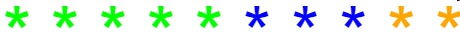What is the ratio of the blue stars to the orange stars?
 a. 3 to 2 c. 5 to 3 b. 3 to 5 d. 2 to 3

3.What is the ratio of green stars to orange stars?
 a. 5:2 c. 2:5 b. 5:10 d. 5:7

Multiple Response
Identify one or more choices that best complete the statement or answer the question.

4.

Which picture(s) show a ratio of 1:2?
 a.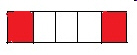c.b.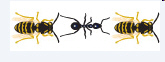d.5.What is the ratio of bees to the total insects?  (There can be more than one answer.)
 a. 6 to 10 c. 3:5 b. 6 :10 d. 10:6

6.What is the ratio of ants to bees?
 a. 4 to 6 c. 6 to 4 b. 10 to 4 d. 4:10

7.Which answers show the ratio of unshaded squares to the whole?  (There is more than 1 correct answer.)
 a. 2:8 c. 3:4 b. 6:2 d. 6:8

8.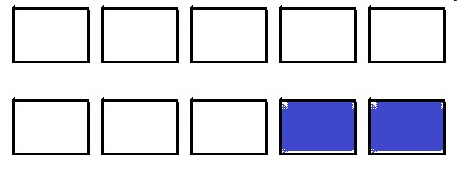a. 2:8 c. 6:2 b. 8:2 d. 2:6

9.What is the ratio of blue spheres to the total number of spheres?
 a. 7 to 3 c. 3 to 7 b. 4 to 3 d. 3 to 4

10.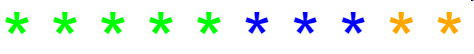What is the ratio of the orange stars to the total number of stars.  Choose all the correct answers.
 a. 2 :10 c. 10:2 b. 10:3 d. 1:5